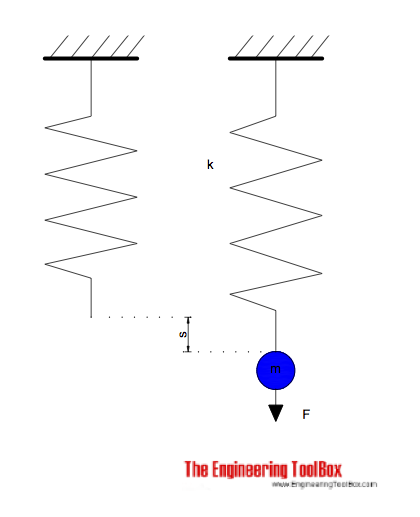Engineering ToolBox - Resources, Tools and Basic Information for Engineering and Design of Technical Applications!

# Hooke's Law

## Hooke's law - force, elongation and spring constant.The force required to extend or compress a spring can be expressed with Hooke's Law as

F = - k s                                    (1)

where

F = force (N)

k  = spring constant (N/m)

s = extension or compression distance (m)

#### Example - Car Suspension

The maximum compression of a car suspension with mass 2000 kg (500 kg on each wheel) shall not exceed 0.1 m.

The force acting on each spring can be calculated using Newton's Second Law

F = (500 kg) (9.81 m/s2)

= 4905 N

= 4.9 kN

The required spring constant for the suspension can be calculated with (1) as

k = - F / s

= - (4905 N) / (- 0.1 m)

= 49050 N/m

= 49 kN/m

Note that this is the value for the static load of the car. You may probably at least double the value for handling dynamic forces, potholes and similar.

### Hook's Law of Elasticity

In the generalized variation of Hooke's law it states that the strain/deformation of an elastic object or material is proportional to the stress applied to it. It may be expressed mathematically as

σ = E ε                              (2)

where

σ = stress (Pa)

E = Young's Modulus of Elasticity (Pa)

ε = strain (m/m)

## Related Topics

• ### Mechanics

Forces, acceleration, displacement, vectors, motion, momentum, energy of objects and more.
• ### Statics

Loads - forces and torque, beams and columns.

## Related Documents

• ### Bolt Stretching and Tensile Stress

Tensile stress and Hooke's Law.
• ### Harmonic Oscillator

A simple harmonic oscillator.
• ### Metals and Alloys - Young's Modulus of Elasticity

Elastic properties and Young's modulus for metals and alloys like cast iron, carbon steel and more.
• ### Speed of Sound - Equations

Calculate the speed of sound (the sonic velocity) in gases, fluids or solids.
• ### Stress in Thick-Walled Cylinders or Tubes

Radial and tangential stress in thick-walled cylinders or tubes with closed ends - with internal and external pressure.
• ### Stress, Strain and Young's Modulus

Stress is force per unit area - strain is the deformation of a solid due to stress.

Weight rating of threaded hanger rods.

## Engineering ToolBox - SketchUp Extension - Online 3D modeling!

Add standard and customized parametric components - like flange beams, lumbers, piping, stairs and more - to your Sketchup model with the Engineering ToolBox - SketchUp Extension - enabled for use with older versions of the amazing SketchUp Make and the newer "up to date" SketchUp Pro . Add the Engineering ToolBox extension to your SketchUp Make/Pro from the Extension Warehouse !

We don't collect information from our users. More about

## Citation

• The Engineering ToolBox (2013). Hooke's Law. [online] Available at: https://www.engineeringtoolbox.com/hookes-law-force-spring-constant-d_1853.html [Accessed Day Month Year].

Modify the access date according your visit.

9.27.11